Browse by Topic

# 13 Ways of Looking at the Number 13

Why are we releasing Version 13 of Mathematica?

As a math and science company, Wolfram doesn’t fear two-digit number integers, especially that particular one between 12 and 14. That is to say, we don’t have triskaidekaphobia, not on Friday the 13th or any other day of the year.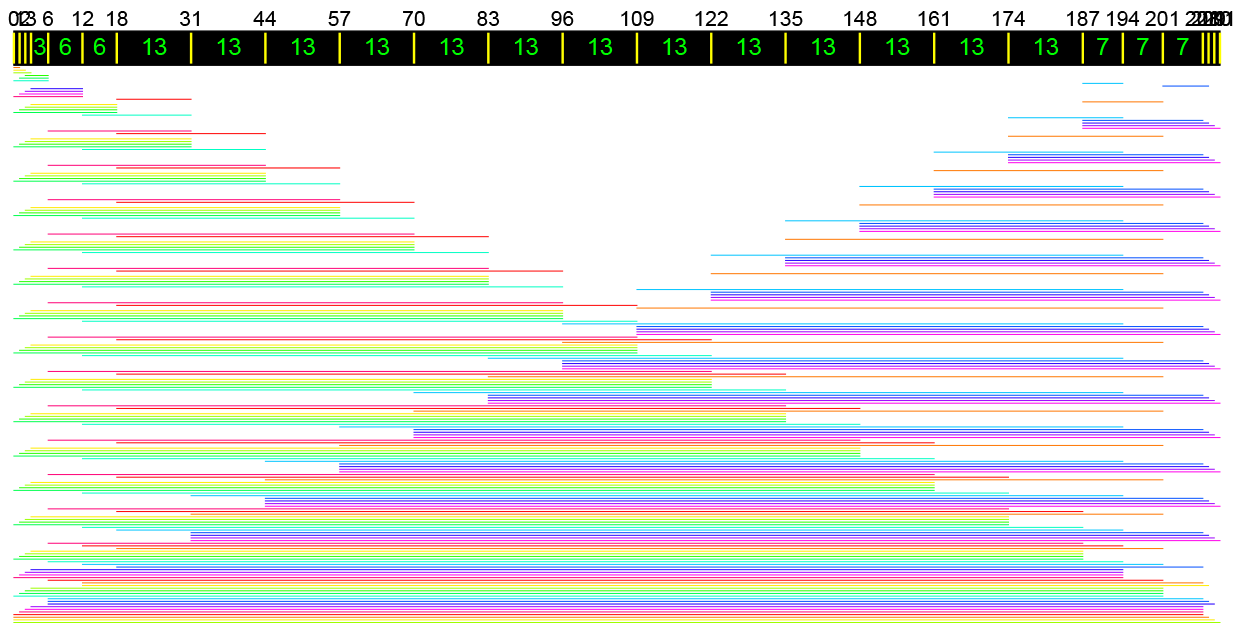Many nice things can be said about 13 beyond it being a ternary repdigit 1113 or the answer to Prime. It’s one of just three known Wilson primes because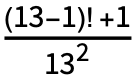= 2 834 329, an integer, and is also the fifth Mersenne prime exponent: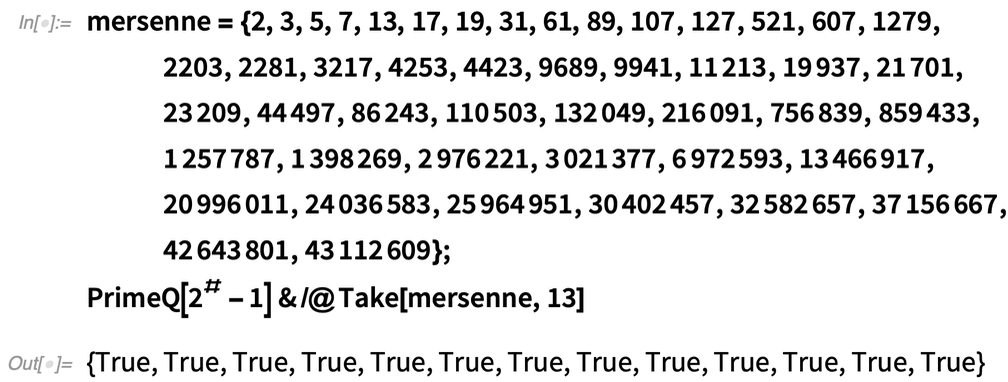✕

But 13 has much more going for it than just being a prime number, and here are 13 reasons why.

1. First, 13 is part of many linear recurrences. For example, 13 is a Fibonacci number where the limit of ratios between values goes to the golden ratio, phi, Φ2 = Φ + 1: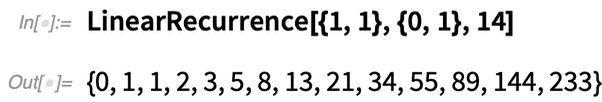✕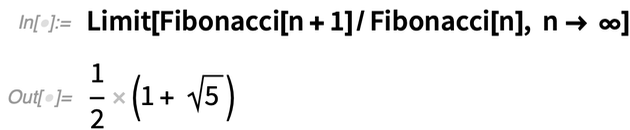✕

The fifth substitution in the Ammann chair tiling thus has 13 chairs: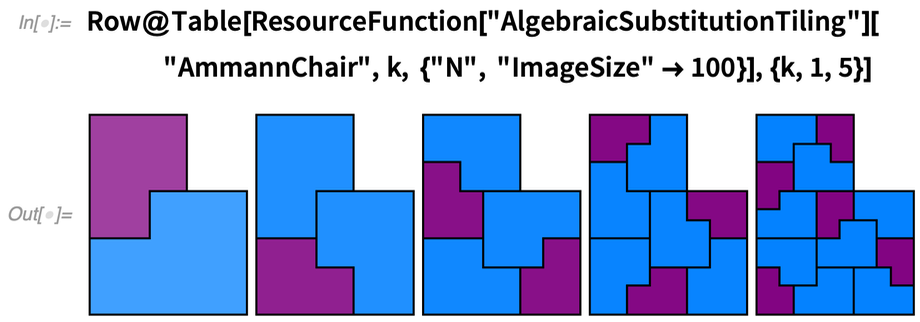✕

Thirteen is also a tribonacci number, a term coined by Mark Feinberg at the age of 14. The ratio between terms goes to the tribonacci constant, t3 = t2 + t + 1: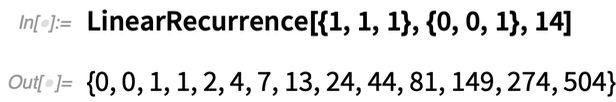✕

Tribonacci numbers can be thought of as binary sequences without 000: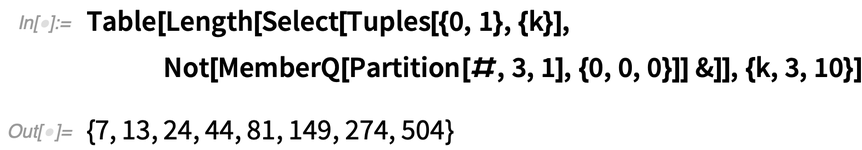✕

2. Thirteen is also a Narayana cow number, based on psi, Ψ3 = Ψ2 + 1, the supergolden ratio: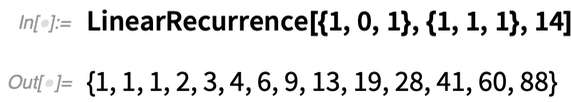✕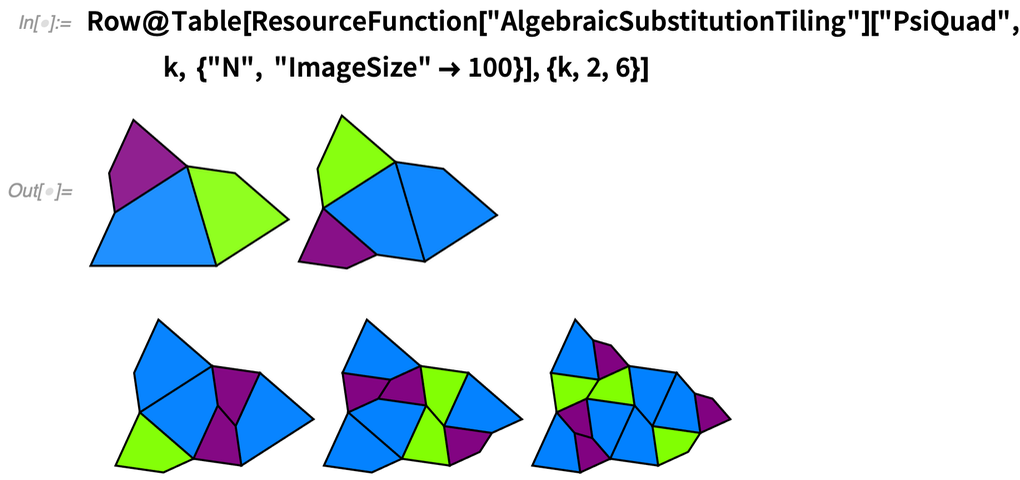✕

3. There are 13 Archimedean solids, in which both the golden ratio and tribonacci constant feature prominently. Similarly, there are 13 Archimedean duals, all of which make fair dice: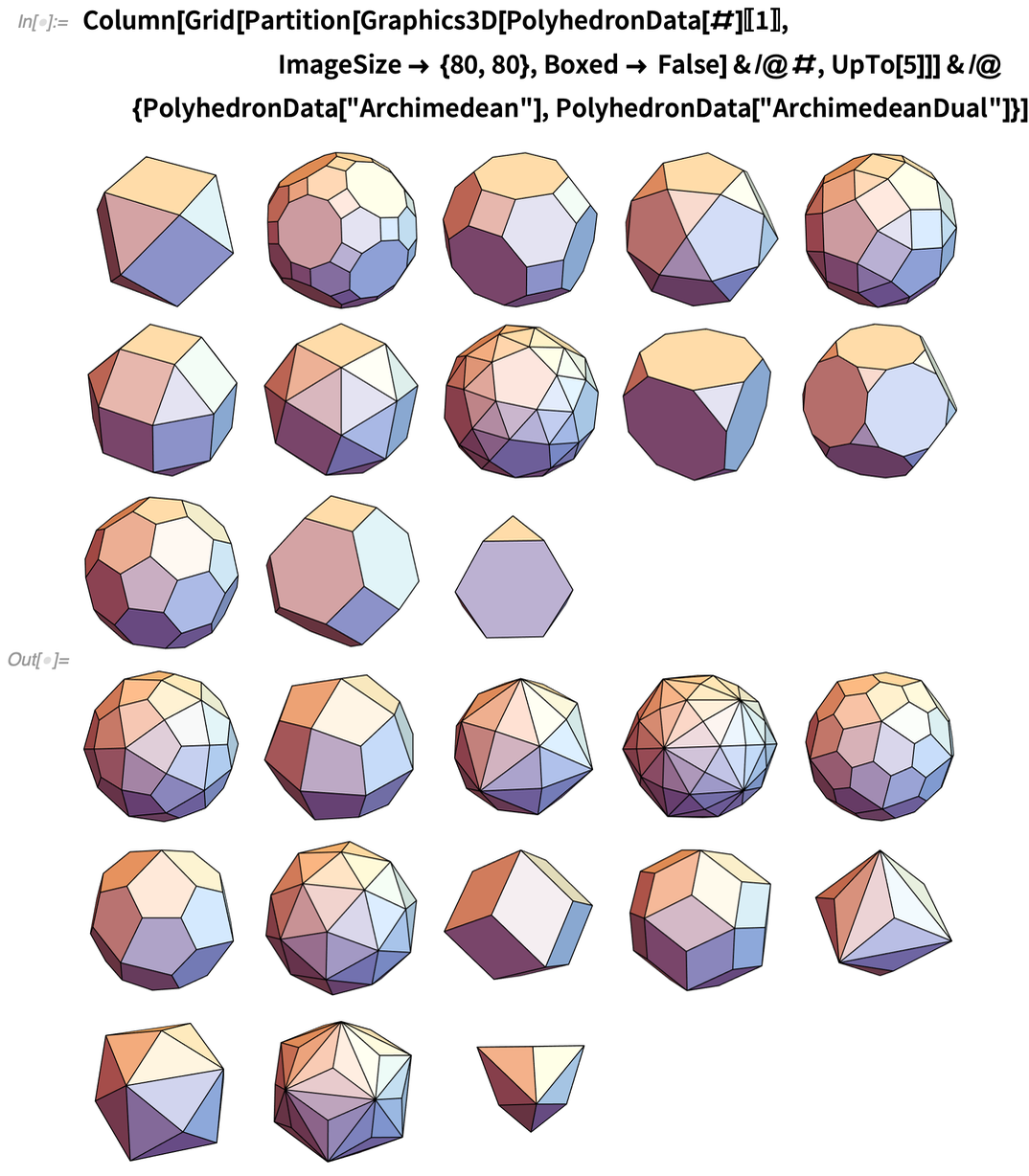✕

4. As a number of form n2 + (n + 1)2, 13 is a centered square number: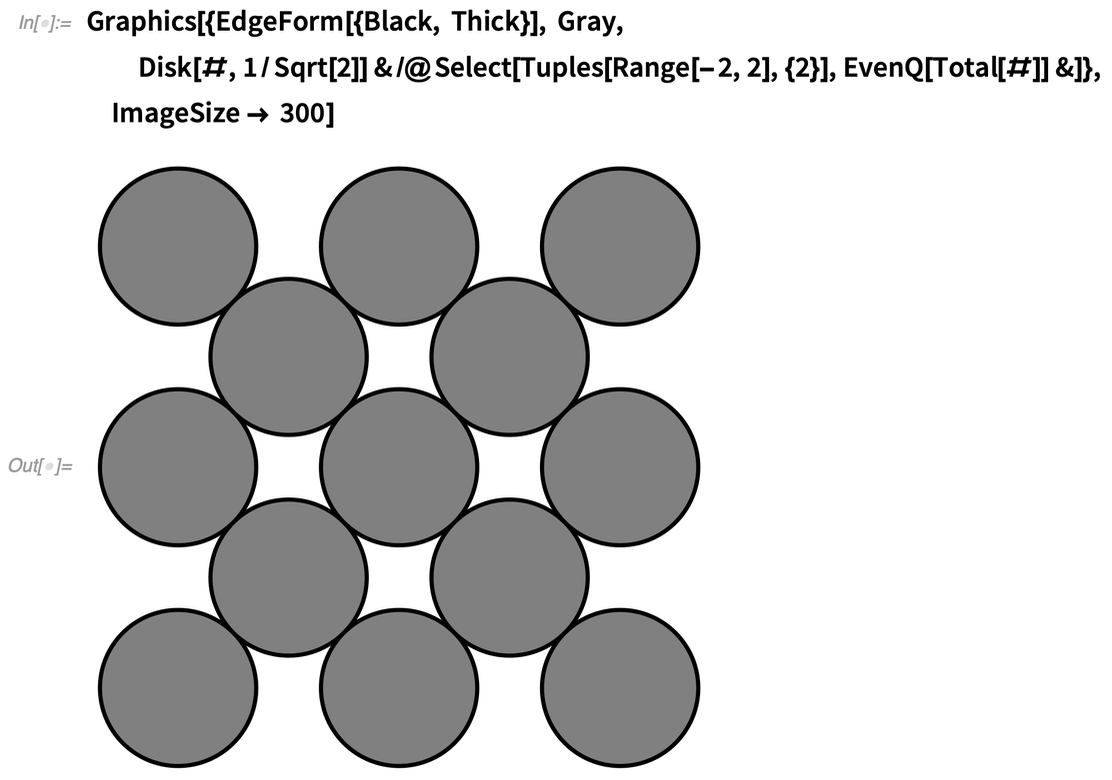✕

5. A combination lock with three buttons has 13 combinations where every button is pressed once, making it the third Fubini, or ordered Bell, number: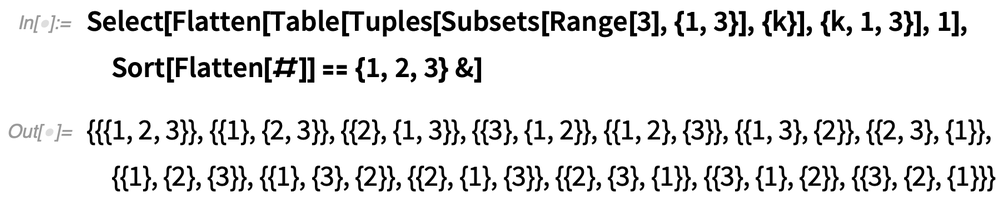✕

6. Balanced ternary notation allows three weights and a two-pan scale to measure weights 1 to 13: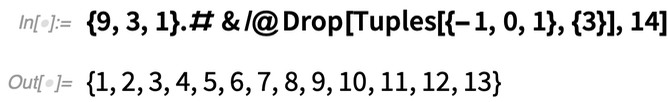✕

7. The remainder graph of 13 has the following form. To use it, pick a number like 2,522. Then start at 0 and follow 2 blue, 1 red, 5 blue, 1 red, 2 blue, 1 red, 2 blue to find the remainder of dividing 2,522 by 13. This works for any positive integer: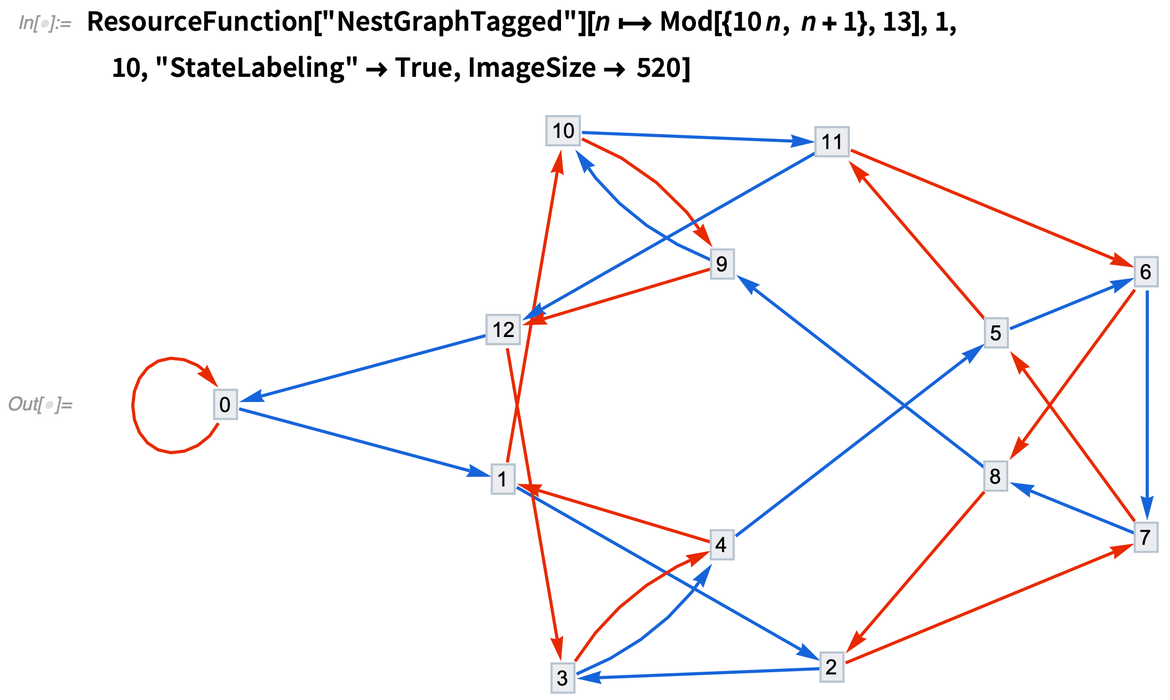✕

8. Using steps of N, E, NE or (1,0), (0,1), (1,1), there are 13 ways to get from (0,0) to (2,2), part of a combinatorial sequence known as the central Delannoy numbers: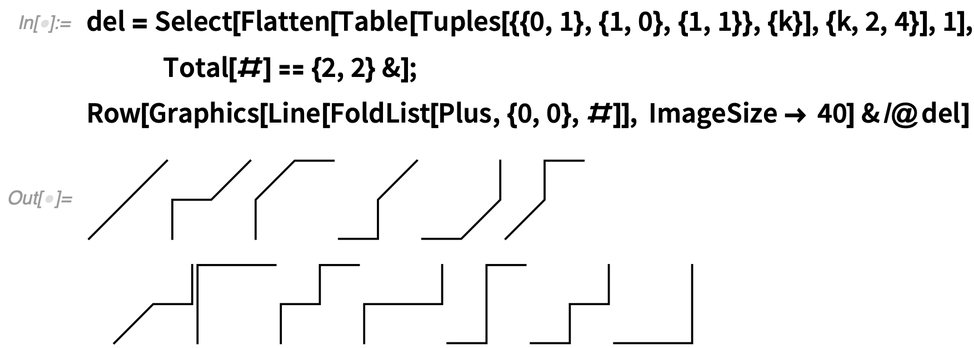✕

These values can be calculated with Legendre polynomials: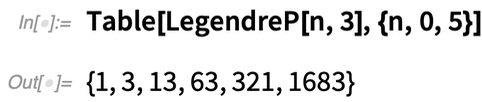✕

9. There are 13 books in Euclid’s Elements. For example, Book I, Proposition 1 discusses the making of an equilateral triangle: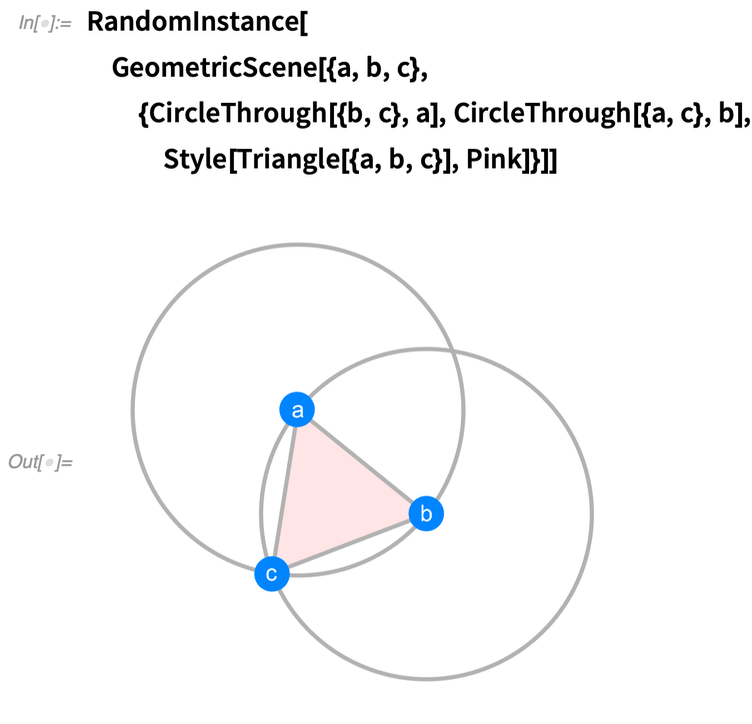✕

10. A sparse ruler of length n allows all integer distances up to n to be measured with a minimal number of marks. The sparse ruler for length 211 uses 26 marks and features 13 spacings of 13: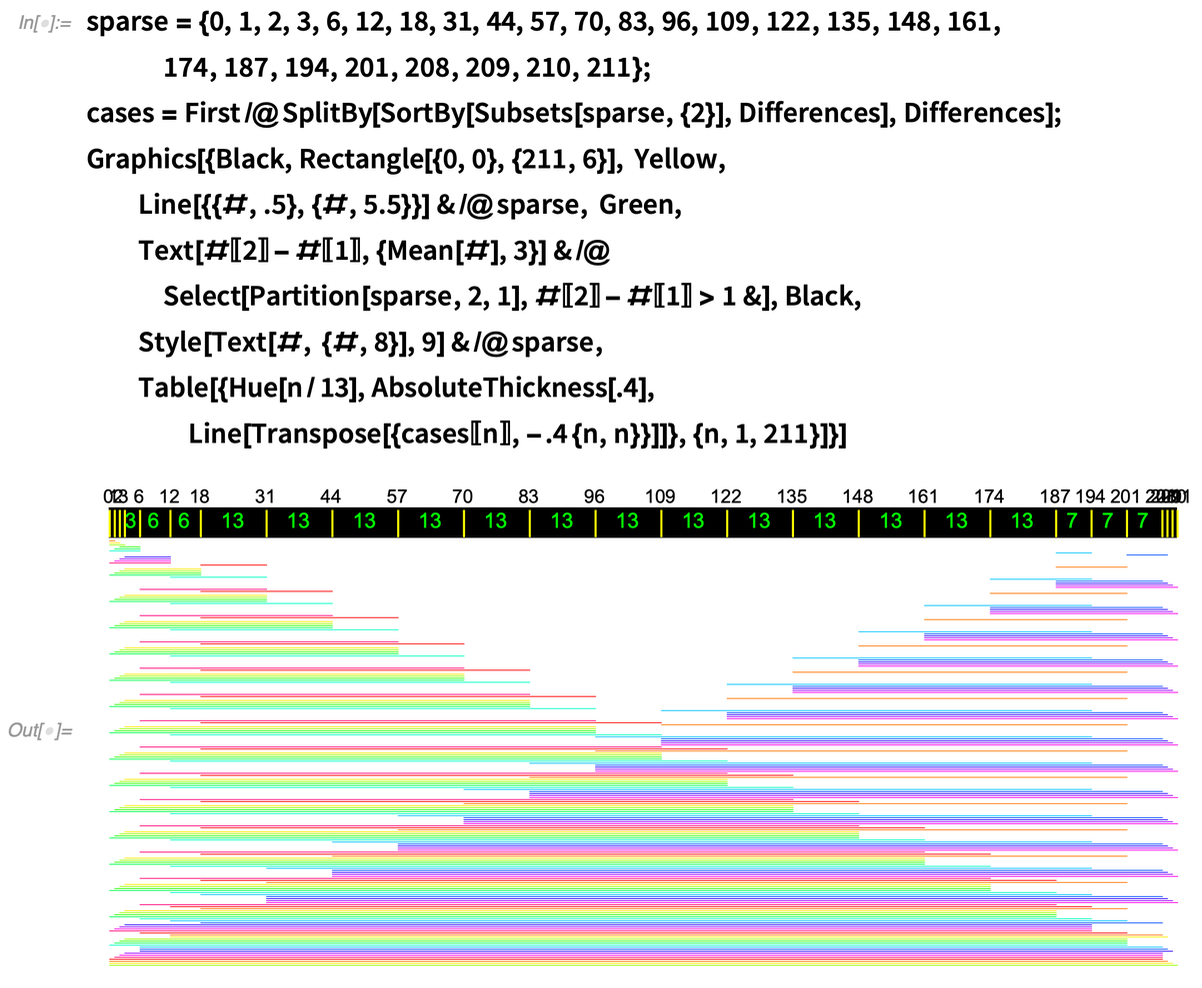✕

11. The BioSequence for the string "THIRTEEN" is as follows: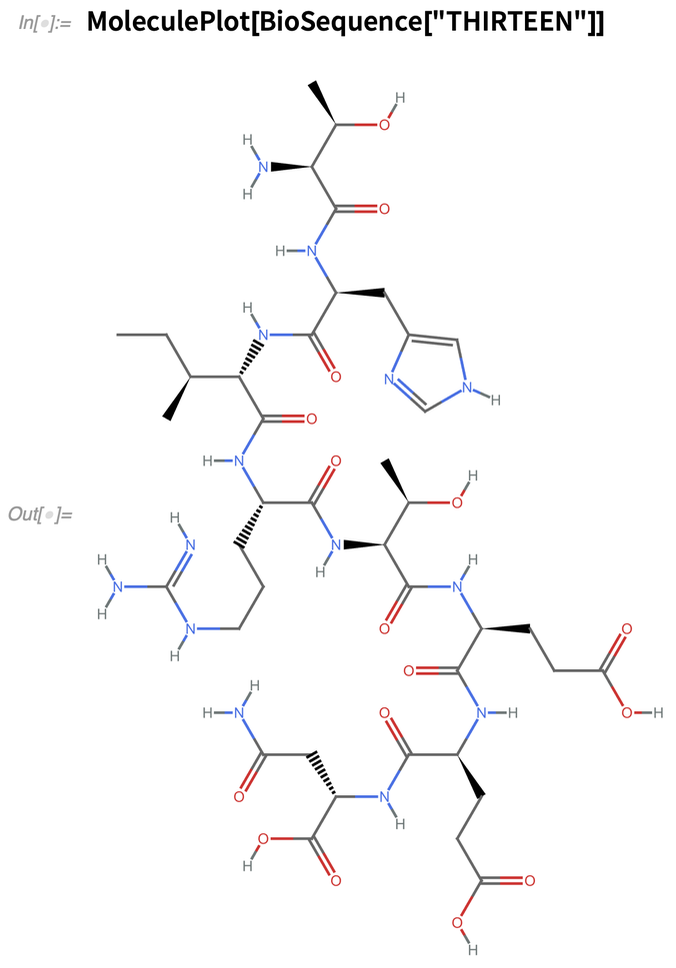✕

12. Books like An Elementary Introduction to the Wolfram Language use ISBN-13 numbers: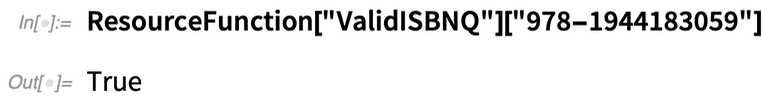✕

13. Finally, for obscuring text in a 26-letter alphabet, the standard is the ROT13 Caesar cipher that rotates each letter forward 13 places: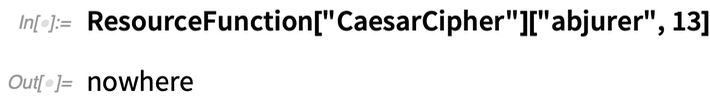✕

This allows us to finish this article with an obscure piece of code: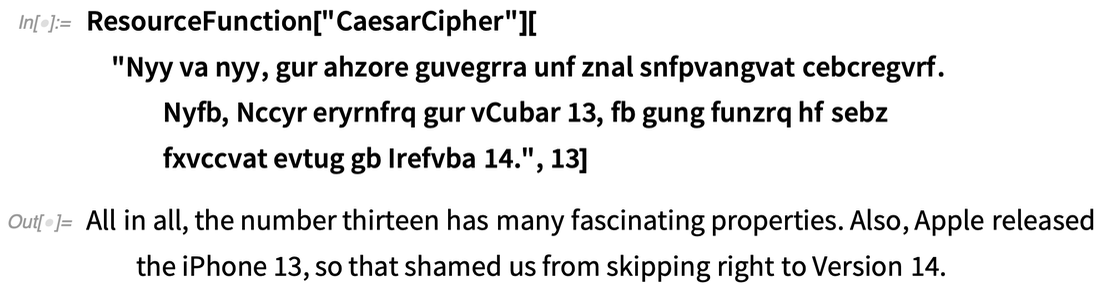✕